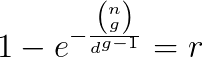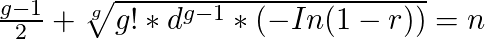## Friday, 23 June 2017

### More Than 2 People Sharing The Same Birthday

There are very few coding examples out there when it comes to calculating the probability of more than 2 people sharing the same birthday. To make this work I have used this Poisson approximation equation, in generalised form this equation looks like this:

n = random people
g = pairs
d = days
r = probabilityHere is the source code:
```  public static double ProbabilityOfPair(int numOfRandomInstances, int uniquePossibilities, int numOfPairs = 2)
{
if (numOfPairs < 2)
throw new Exception("Cannot have less than two pairs");

if (numOfRandomInstances < 1 || uniquePossibilities < 1)
throw new Exception("Number of random instances or unique possibilities needs to be greater than 0");

double bionomial = calcBinomialCoefficient(numOfRandomInstances, numOfPairs);
double totalPairPossibilities = Math.Pow(uniquePossibilities, numOfPairs - 1);
return 1 - Math.Exp(-(bionomial / totalPairPossibilities));
}

static double calcBinomialCoefficient(double n, double k)
{
if (k > n)
throw new Exception("n must be greater than k");

double result = 1;
for(int i = 1; i <= k; i++)
{
result *= n - (k - i);
result /= i;
}
return result;
}
```

### Birthday Problem Reverse

Now what do you do if you want to reverse the birthday problem and instead get back number of random people required to reach certain probability? For example, instead of this:
`  ProbabilityOfPair(23, 365, 2) // returns 0.5000017521827107`
You would like to do something like this:
```  FindNumOfRandomInstancesRequired(365, 2, 0.50) // returns 23
```

To begin with I’ve used ProbabilityOfPair function and just did a linear search. This did not scale very well at all, so I have decided to use binary search algorithm instead. This has worked very well, however it still felt inefficient. So, I have decided to rearrange the above equation, this is what I have ended up with:

n = random people
g = pairs
d = days
r = probabilityHere is the source code:
```  public static int FindNumOfRandomInstancesRequired(int uniquePossibilities, int numOfPairs, double requiredProbability)
{
if (requiredProbability < 0.00 || requiredProbability >= 1.00)
throw new Exception("Required probability must be between 0.00 and 1.00");

double inverse = -Math.Log(1.0 - requiredProbability);
double bionomialFact = factorial(numOfPairs);
double totalPairPossibilities = Math.Pow(uniquePossibilities, numOfPairs - 1);
double precision = (numOfPairs - 1.0) / 2.0;
double numOfRandomInstancesRequired = Math.Pow(bionomialFact * totalPairPossibilities * inverse, 1.0 / numOfPairs) + precision;

return (int)Math.Round(numOfRandomInstancesRequired, 0, MidpointRounding.AwayFromZero);
}

static int factorial(int i)
{
if (i <= 1)
return 1;
return i * factorial(i - 1);
}
```

### Full Source Code (licensed under the GNU licence v3.0)

```  public class BirthdayProblemPoissonApprox
{
/// <summary>
/// Generalised birthday problem probability calculation using poisson approximation.
/// </summary>
/// <example>
///  ProbabilityOfPair(23, 365), returns 0.5000017521827107
///  ProbabilityOfPair(30, 365, 3), returns 0.030015086543320635
/// </example>
/// <param name="numOfRandomInstances">Number of random instances in the group e.g. number of random people in the group</param>
/// <param name="uniquePossibilities">Number of unique possibilities that random instances can fit into e.g. number of unique days</param>
/// <param name="numOfPairs">Number of pairs</param>
/// <see cref="https://en.wikipedia.org/wiki/Birthday_problem"/>
/// <remarks>To make this scale beyond 2 pairs, I have used equations from here: https://math.stackexchange.com/questions/25876/probability-of-3-people-in-a-room-of-30-having-the-same-birthday </remarks>
/// <returns>Probability of pair</returns>
public static double ProbabilityOfPair(int numOfRandomInstances, int uniquePossibilities, int numOfPairs = 2)
{
if (numOfPairs < 2)
throw new Exception("Cannot have less than two pairs");

if (numOfRandomInstances < 1 || uniquePossibilities < 1)
throw new Exception("Number of random instances or unique possibilities needs to be greater than 0");

double bionomial = calcBinomialCoefficient(numOfRandomInstances, numOfPairs);
double totalPairPossibilities = Math.Pow(uniquePossibilities, numOfPairs - 1);
return 1 - Math.Exp(-(bionomial / totalPairPossibilities));
}
/// <summary>
/// Generalised birthday problem reversed, find number of random instances required to fit certain probability.
/// </summary>
/// <example>
/// FindNumOfRandomInstancesRequired(365, 2, 0.50), returns 23
/// FindNumOfRandomInstancesRequired(365, 3, 0.03), returns 30
/// </example>
/// <param name="uniquePossibilities">Number of unique possibilities that random instances can fit into e.g. number of unique days</param>
/// <param name="numOfPairs">Number of pairs</param>
/// <param name="requiredProbability">Probability that must be reached</param>
/// <returns>Number of random instances required</returns>
public static int FindNumOfRandomInstancesRequired(int uniquePossibilities, int numOfPairs, double requiredProbability)
{
if (requiredProbability < 0.00 || requiredProbability >= 1.00)
throw new Exception("Required probability must be between 0.00 and 1.00");

double inverse = -Math.Log(1.0 - requiredProbability);
double bionomialFact = factorial(numOfPairs);
double totalPairPossibilities = Math.Pow(uniquePossibilities, numOfPairs - 1);
double precision = (numOfPairs - 1.0) / 2.0;
double numOfRandomInstancesRequired = Math.Pow(bionomialFact * totalPairPossibilities * inverse, 1.0 / numOfPairs) + precision;

return (int)Math.Round(numOfRandomInstancesRequired, 0, MidpointRounding.AwayFromZero);
}

/// <summary>
/// Calculates efficiently number of possible combinations, "n choose k".
/// </summary>
/// <example>For example, suppose you have a deck of 5 cards (n = 5) and you want to know how many different hands of 3 cards you can make (k = 3). If you number the cards 1 through 5, then you will get 10 possible combinations.</example>
/// <param name="n">Total set of items</param>
/// <param name="k">Number of items to pick from n</param>
/// <see cref="http://www.vb-helper.com/howto_calculate_n_choose_k.html"/>
/// <returns></returns>
static double calcBinomialCoefficient(double n, double k)
{
if (k > n)
throw new Exception("n must be greater than k");

double result = 1;
for(int i = 1; i <= k; i++)
{
result *= n - (k - i);
result /= i;
}
return result;
}

static int factorial(int i)
{
if (i <= 1)
return 1;
return i * factorial(i - 1);
}
}
```

## Sunday, 11 June 2017

### Repeating String Pattern Search

In this post I am going to introduce basic repeating string pattern search algorithm that I have written for fun. This algorithm’s average running time is Θ(N), worst running time is O(N^2) (see analysis below). This algorithm takes ~1 minute to search 10 million digits of Pi.

#### Example usage

```
string raw = "091283091283091831093810933";
Dictionary<string, List<int>> patterns = DiscoverPatterns(raw);
foreach (KeyValuePair<string, List<int>> pattern in patterns)
{
Console.WriteLine(\$"Pattern: {pattern.Key}, Indexes: {String.Join(", ", pattern.Value)}");
}
```

#### Example output

Pattern: 091283091, Indexes: 0, 6
Pattern: 1093, Indexes: 17, 22

### Source Code (licensed under the GNU licence v3.0)

```
public static Dictionary<string, List<int>> DiscoverPatterns(string raw, int patternSizeMin = 2)
{
if (patternSizeMin < 1)
throw new Exception("Minimum pattern size should not be less than 1.");

Dictionary<string, HashSet<int>> initialPatternSearch = new Dictionary<string, HashSet<int>>();
Dictionary<string, HashSet<int>> discoveredPatterns = new Dictionary<string, HashSet<int>>();

int numberOfInitialSearches = raw.Length - (patternSizeMin - 1);
for (int i = 0; i < numberOfInitialSearches; i++)
{
string pattern = raw.Substring(i, patternSizeMin);

HashSet<int> patternIndexes;
if (initialPatternSearch.TryGetValue(pattern, out patternIndexes))
{
discoveredPatterns[pattern] = patternIndexes;
}
else
{
patternIndexes = new HashSet<int>();
initialPatternSearch.Add(pattern, patternIndexes);
}

patternIndexes.Add(i);
}

initialPatternSearch.Clear();

Dictionary<string, HashSet<int>> basePatterns = discoveredPatterns;
do
{
Dictionary<string, HashSet<int>> superPatterns = discoverSuperPatterns(raw, ++patternSizeMin, basePatterns);

foreach (KeyValuePair<string, HashSet<int>> superPattern in superPatterns)
{
string basePattern = superPattern.Key.Substring(0, patternSizeMin - 1);
discoveredPatterns.Remove(basePattern);

string baseInnerPattern = superPattern.Key.Substring(1, patternSizeMin - 1);
discoveredPatterns.Remove(baseInnerPattern);

discoveredPatterns.Add(superPattern.Key, superPattern.Value);
}

basePatterns = superPatterns;
} while (basePatterns.Count != 0);

return discoveredPatterns.OrderByDescending(pattern => pattern.Key.Length).ToDictionary(pattern => pattern.Key, x => x.Value.ToList());
}

private static Dictionary<string, HashSet<int>> discoverSuperPatterns(string raw, int patternSize, Dictionary<string, HashSet<int>> basePatterns)
{
Dictionary<string, HashSet<int>> initialSuperPatternSearch = new Dictionary<string, HashSet<int>>();
Dictionary<string, HashSet<int>> discoveredSuperPatterns = new Dictionary<string, HashSet<int>>();

foreach (KeyValuePair<string, HashSet<int>> basePattern in basePatterns)
{
foreach (int patternIndex in basePattern.Value)
{
if (raw.Length >= (patternIndex + patternSize))
{
string superPattern = raw.Substring(patternIndex, patternSize);

HashSet<int> superPatternIndexes;
if (initialSuperPatternSearch.TryGetValue(superPattern, out superPatternIndexes))
{
discoveredSuperPatterns[superPattern] = superPatternIndexes;
}
else
{
superPatternIndexes = new HashSet<int>();
initialSuperPatternSearch.Add(superPattern, superPatternIndexes);
}

superPatternIndexes.Add(patternIndex);
}
}
}

return discoveredSuperPatterns;
}
```

### Analysis

#### Worst Case

If you provide input such as 010101…01 then running time will be ((N(N-1))/2)-4, this gives us a triangular number. This is because this input is just one big continuous pattern and it’s discovering patterns one character at the time i.e. (N-1)+(N-2)+(N-3)…+(N-(N-2)). If we drop all terms that grow slowly we end up with O(N^2).

#### Average Case

If input is not a big continuous pattern but has many small sub patterns then running time will be X*N. If we drop all terms that grow slowly we end up with average running time Θ(N).